#### How to Use Hlookup Function in Excel

In this lesson, you will learn HLOOKUP function.

## Hlookup function syntax

HLOOKUP works similarly to the VLOOKUP function. HLOOKUP is a lookup and reference function. This function retrieves data from the table horizontally. Most of the tables in Excel, create a vertical, so this feature is rarely used.

Despite it, I think it’s worth it to know HLOOKUP. HLOOKUP function has the following form:

=HLOOKUP(reference,array,row_number,row)

In simple terms it can be assumed that the individual components of this function:

=VLOOKUP(what,where,in which row,true/false)

The last part of the formula is very important:

• True is an approximate match,
• False is the exact value.

I prepared a table of sales.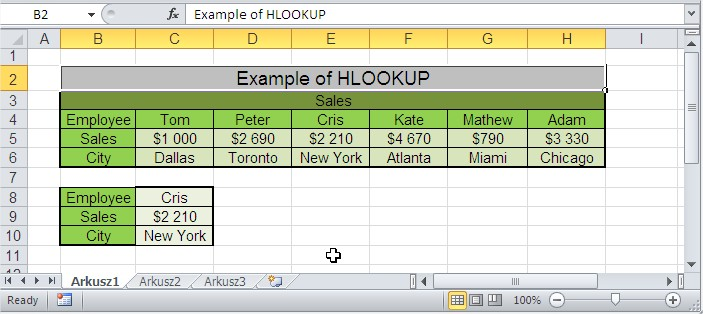You’ll learn from the above table, as HLOOKUP works.

With the name of the employee when you appear all the data on it that contains the table. At the beginning of the selection of employees could use the drop-down list.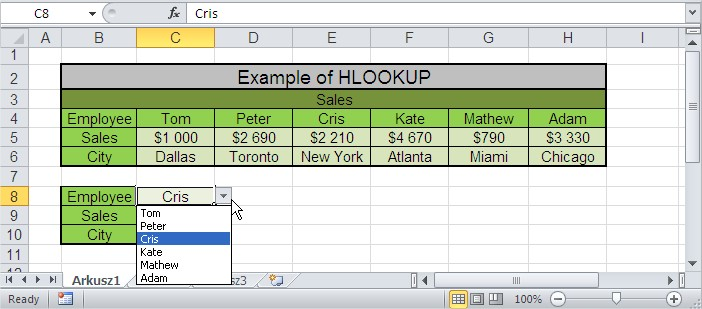In the sales, type the formula. It is:

=HLOOKUP(C7,C3:H5,2,FALSE)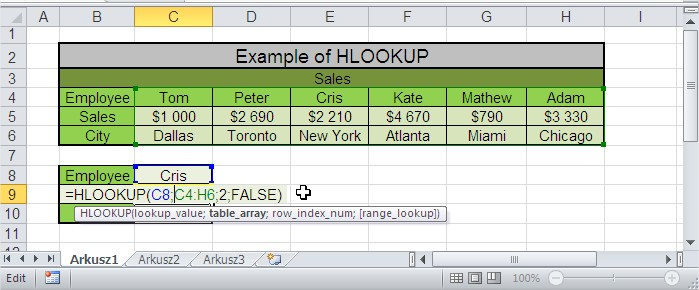Drag the formula in the City field. In this way, HLOOKUP searches for data from the table and selecting the employee name appears in the sale and the city. This of course is just a simple example.

HLOOKUP is applicable especially in large tables, where the search data is no longer so simple. Examples of such data table to report sales or payroll.

Let’s analyze some more business examples on how to use HLOOKUP function in Excel.

## Hlookup function examples

### Example 1: Look for Price Horizontally with HLOOKUP

In this example, we have laid out the price in a horizontal form, and we do not know the price of motorcycle, so we decide to use the HLOOKUP function in Excel to find out the price.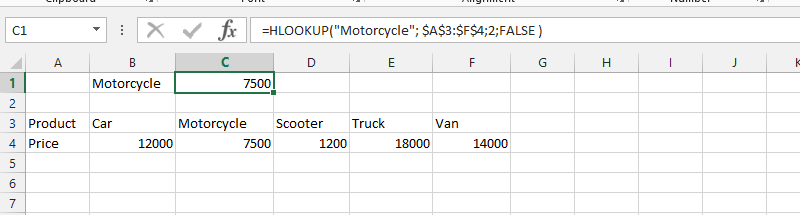### Example 2: Finding Price Vertically with HLOOKUP

In this example, we have already established a business, and our products’ prices have been dynamic. We’d know how many times we have changed the prices. Now, we want to know how much our customers paid for purchasing a car, when we changed the price for a second time.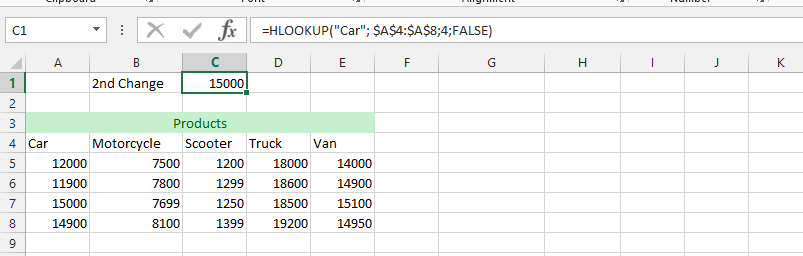### Example 3: HLOOKUP Finds Email Address

We have a lot of employees/customers, and we would like to find the email address of one of them. We’d know the name of the person, but we do not know his or her email address, and we do not write the employees/customers’ names in the same column, but we’d spread them out. This is another place HLOOKUP can be a very handy function in Excel, even though they are other functions that could do the same thing.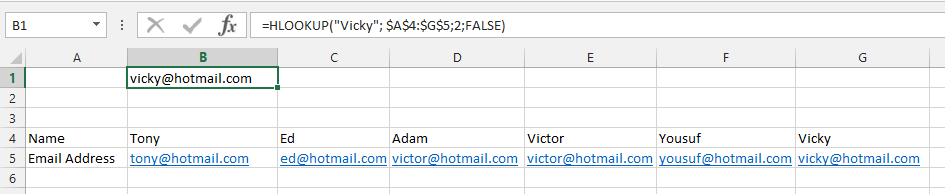### Example 4: Locating how Much a Customer Owns

In this example, we’d have many customers, but some of them are owing the company money. We’d know the name of the person, but we do not know the amount that the person is owning. In this circumstances, we are using HLOOKUP function to find the amount of money that Charles is owning.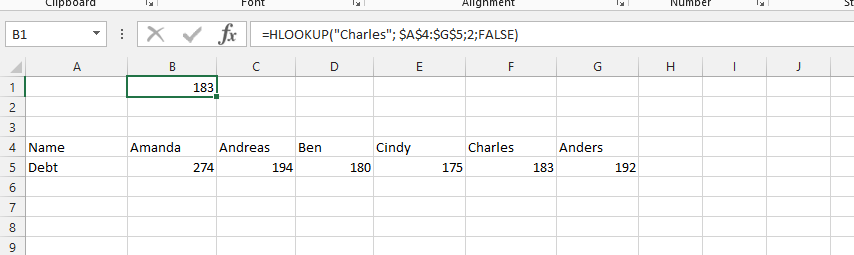### Example 5: Did Anybody Paid in 500?

We have understanding that there have been information about different income into the company, but there is a little misunderstanding. We’d have 500 in the account, and we do not know if it was paid by a customer or if someone else have paid the money, which lead to us looking for it with the help of HLOOKUP function. We’d go to the Excel, where customers’ payments are saved. It is important that we are looking for is on top, otherwise the function would not work.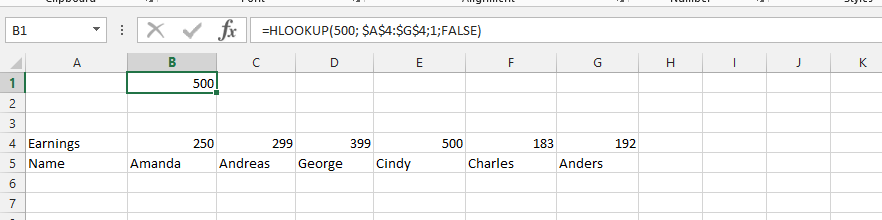### Example 6: Dealing With the Name Error

There will be circumstances, where the name error is visible on the Excel, but this is not something that should cause any alarm. The reason that the error exists is that we do not add symbol “” to the name. In this example, the only thing needed is just to add the “” to the name (one (“) in the beginning of the name, and the second (“) would be right after the name).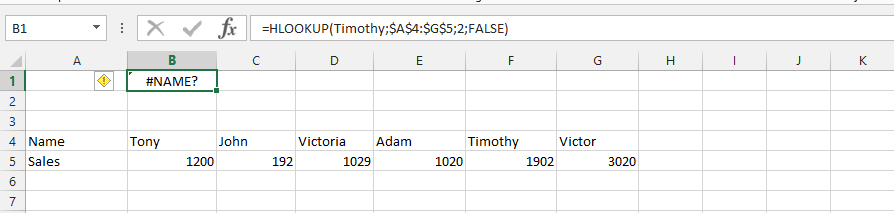### Example 7: How to Deal with N/A Error

In this example, we will look into the possibility of N/A error showing when using the HLOOKUP function, and how we will easily deal with it. In the error, there are many reasons these errors could be showing, it could be that what we are looking for is not in the file, but it can also be that the term is not in the first role, which is where the HLOOKUP function firstly used to do everything it needs to do.

One of the best way to deal with this is to look for something that is in the first row, and if what you are looking for is not in the Excel spreadsheet, then it is not necessary for it to be a problem.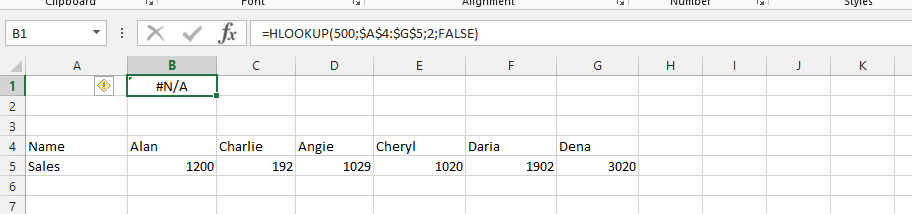### Example 8: Locating Employees’ Telephone Number

In this example, we’d have ten employees, but we’d like to rather use the HLOOKUP function, to simplifies and get the accurate telephone number of the employee, without any risk of getting the telephone number wrong. We have used the HLOOKUP to find the telephone number to the employee, whom we are looking for.

See also  How To Use The Index And Match Functions In Excel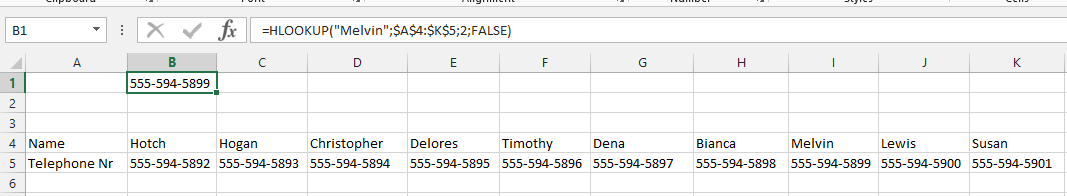### Example 9: Finding Address to Employee

In this example, we need to send out paycheck for a specific employee who did not get her paycheck, because she was on vacation. This is where the HLOOKUP function can be useful among other functions that Excel have provided.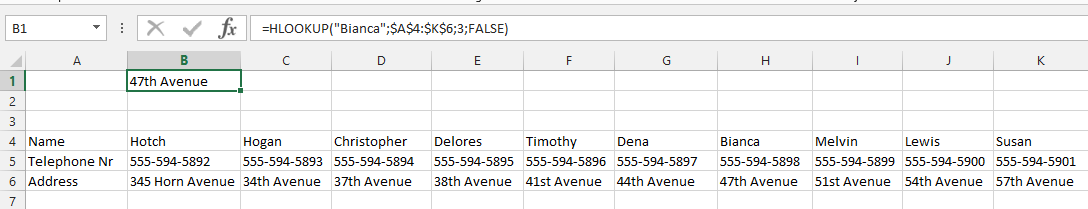### Example 10: Confirming Payment

With this function, it is very easy to know if there is a specific value under a name, under the circumstances of having a customer who said that he has paid an amount, but the timing were too close, and the customer have gone from one employee to the other. The customer says that he paid too much, and we would like to confirm it, so we could pay back the money to the customer.

This is one of the places, where HLOOKUP is very useful, under certain circumstances, because VLOOKUP function might be more appropriate under other circumstances.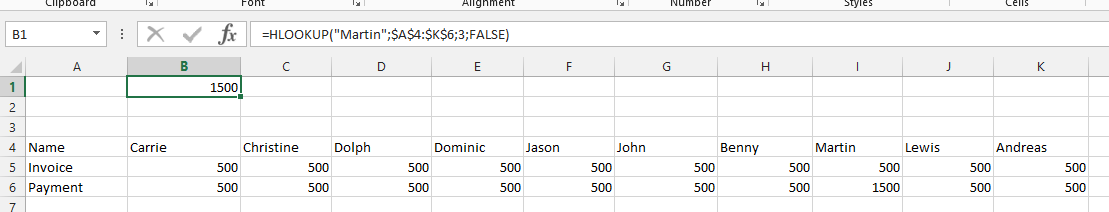## Key Takeaways

• HLOOKUP is a lookup and reference function that retrieves data from a table horizontally.
• The HLOOKUP function has the following syntax: =HLOOKUP(reference,array,row_number,row).
• The reference is the value you are looking for in the table.
• The array is the range of cells that contains the table.
• The row_number is the row number of the cell that contains the value you are looking for.
• The row is a logical value that determines whether an exact match or an approximate match is returned.

## FAQ

• Q: What is the difference between HLOOKUP and VLOOKUP?
• A: HLOOKUP searches for a value in a table horizontally, while VLOOKUP searches for a value in a table vertically.
• Q: What are some examples of how HLOOKUP can be used in Excel?
• A: HLOOKUP can be used to find a customer’s phone number, an employee’s salary, or the price of a product.